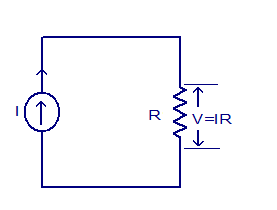## Audio

[Audio][slideshow]

## Transimpedance amplifier / current to voltage converter.

The transimpedance amplifier is a circuit that converts current to a proportional voltage. It is also termed as current to voltage converter or simple I to V converter. The transimpedance amplifier has ideally zero input resistance and low output resistance. The transconductance amplifier has a lot of applications in day to day electronics. For example, converting to current detected by a photo diode into a proportional voltage, digital to analog converter (DAC) applications etc. Current to voltage converters are of two types, passive and active.

### Passive current to voltage converter.

As the name indicates, only passive elements are used for implementing the circuit. The circuit diagram of a passive current to voltage converter is shown below. Here the resistor R converts the current I from the current source into a proportional voltage across R itself. The mechanism follows Ohm’s law (V= IR).Passive current to voltage converter.

Anyway the passive current to voltage converter has many limitations. For the proper operation, the input current source and the output load must be ideal. That means the output load resistance must be close to infinity, the current from the current source must be independent of the voltage created across load resistor ( current source must have unlimited capacity) and also the current source must have infinite internal resistance. These conditions are practically impossible to attain and so passive current to voltage converters are seldom used in practical applications.

### Active current to voltage converter ( transimpedance amplifier).

An active current to voltage converter (transimpedance amplifier) is based on active elements like BJTs, FETs or an opamp. Transimpedance amplifier using opamp is the commonly used one. The circuit diagram of an opamp based current to voltage converter is shown below.Active transimpedance amplifier circuit

In the circuit diagram V+ and V- are the voltage sources for the opamp. Rf is the feedback resistor and RL is the load resistor.

#### Expression for the output voltage.

The equation for the output voltage of the opamp based current to voltage converter can be derived as follows. The opamp here is wired in closed loop inverting mode with feedback resistor = Rf. Let iIN be the input current that needs to be converted and Vout be the output voltage of the converter circuit.

The equation for the voltage gain (Av) of an opamp inverting amplifier can be written as,

Av = – Rf/R1.

Therefore Vout = -(Rf/R1) (Vin)……….(1)

Since non inverting input is connected to ground and the ideal opamp has infinite input resistance, we can assume V1 = 0 and V1=V2.

Also i IN = Vin/R1……………………………(2)

Substituting (2) in equation (1) we get

Vout = -(i IN) ( RF)

In simple words, a current to voltage converter using opamp is made by replacing R1 and Vin of an opamp inverting amplifier and replacing it with a current source i IN.

[Lights][grids]